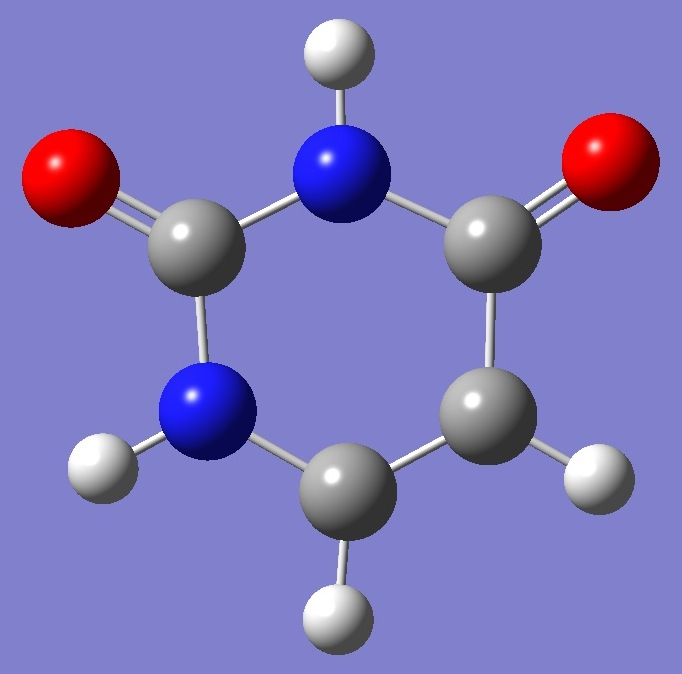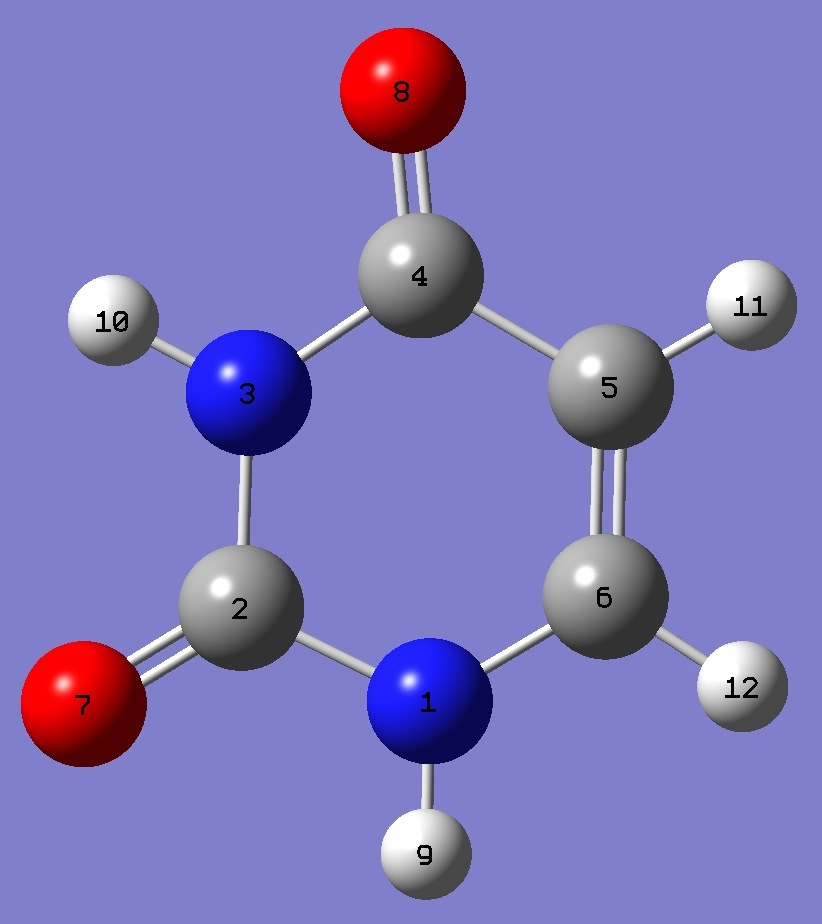UracilNitrogen

Nuclear Quadrupole Coupling Constants

in Uracil

Nitrogen nqcc's in uracil were determined by Vaquero et al. , and by Brünken et al. .  A heavy atom substitution structure was determined by Vaquero et al. .  A best estimate ab initio equilibrium structure was determined by Puzzarini and Barone , and a semi-experimental equilibrium structure by Császár et al. .

Calculation of the 14N nqcc tensors was made here on the reSE molecular structure of Császár et al. .  Calculated and experimental  nqcc's are compared in Tables 1 and 2.  Structure parameters are given in Z-matrix format in Table 3.

Calculation was made with both B3PW91/6-311+G(df,pd) and B3PW91/6-311+G(d,p) models.

In Tables 1 and 2, subscripts a,b,c refer to the principal axes of the inertia tensor; x,y,z to the principal axes of the nqcc tensor.  ETA = (Xxx - Xyy)/Xzz.

RMS is the root mean square difference between calculated and experimental diagonal nqcc's (percentage of the average of the magnitudes of the experimental nqcc's).  RSD is the calibration residual standard deviation model for calculation of nitrogen efg's/nqcc's.

 Table 1.  14N(1) nqcc's in Uracil (MHz).  Calculation was made on the reSE molecular structure with both (1) B3PW91/6-311+G(df,pd) and (2) B3PW91/6-311+G(d,p) models. Calc (1) Calc (2) Expt.  Xaa 1.729 1.800 1.7672(68) Xbb 1.864 1.955 1.9748(83) Xcc - 3.593 - 3.755 - 3.7420(83) |Xab| 0.144 0.163 RMS 0.109 (4.4 %) 0.023 (0.93 %) RSD 0.030 (1.3 %) 0.086 (3.8 %) Xxx 1.637 1.697 Xyy 1.956 2.058 Xzz - 3.593 - 3.755 ETA 0.088 0.096

 Table 2.  14N(3) nqcc's in Uracil (MHz).  Calculation was made on the reSE molecular structure with both (1) B3PW91/6-311+G(df,pd) and (2) B3PW91/6-311+G(d,p) models. Calc (1) Calc (2) Expt.  Xaa 1.860 1.960 1.9161(68) Xbb 1.487 1.540 1.5344(90) Xcc - 3.347 - 3.500 - 3.4505(90) |Xab| 0.154 0.157 RMS 0.073 (3.2 %) 0.038 (1.7 %) RSD 0.030 (1.3 %) 0.086 (3.8 %) Xxx 1.432 1.487 Xyy 1.915 2.013 Xzz - 3.347 - 3.500 ETA 0.144 0.150

 Table 3.  Uracil.  Structure parameters, reSE  (Å and degrees).N  C,1,R1  N,2,R2,1,A1  C,3,R3,2,A2,1,D1,0  C,4,R4,3,A3,2,D2,0  C,1,R5,2,A4,3,D3,0  O,2,R6,1,A5,6,D4,0  O,4,R7,3,A6,2,D5,0  H,1,R8,2,A7,3,D6,0  H,3,R9,4,A8,5,D7,0  H,5,R10,6,A9,1,D8,0  H,6,R11,1,A10,2,D9,0 R1=1.3810  R2=1.3749  R3=1.3991  R4=1.4548  R5=1.3722  R6=1.2101  R7=1.2186  R8=1.0043  R9=1.0083  R10=1.0757  R11=1.0781  A1=113.38  A2=127.97  A3=113.75  A4=123.39  A5=122.78  A6=120.41  A7=115.14  A8=116.41  A9=122.12  A10=115.39  D1=0.  D2=0.  D3=0.  D4=180.  D5=180.  D6=180.  D7=180.  D8=180.  D9=180.

 V.Vaquero, M.E.Sanz, J.C.López, and J.L.Alonso, J.Phys.Chem. A Letters, 111,3443(2007).

 S.Brünken, M.C.McCarthy, P.Thaddeus, P.D.Godfrey, and R.D.Brown, A&A 459,317(2006).

 C.Puzzarini and V.Barone, PCCP, 13,7158(2011).  This work includes also a high level CCSD(T) calculation of the nqcc's.

 A.G.Császár, J.Demaison, and H.D.Rudolph, J.Phys.Chem. A, 119(9),1731(2015).

Pyridine Pyrimidine Quinoline Isoquinoline

2-Pyridone 2-Hydroxypyridine
Thymine

Molecules/Nitrogen

Uracil_2.html